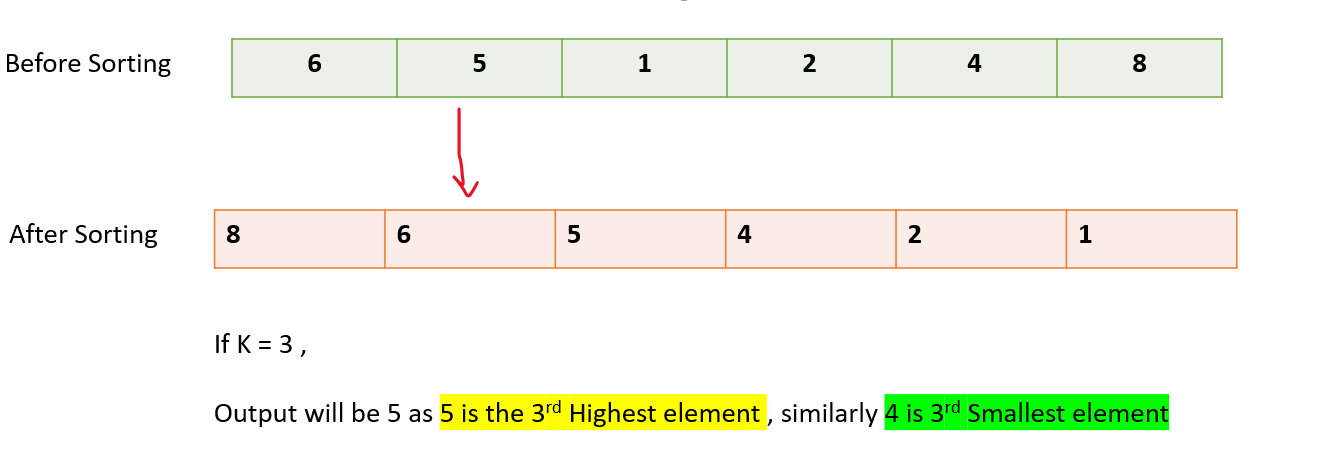# Kth largest/smallest element in an array

Problem Statement: Given an unsorted array, print Kth Largest and Smallest Element from an unsorted array.

Examples:

```Example 1:
Input: Array = [1,2,6,4,5,3] , K = 3
Output: kth largest element = 4, kth smallest element = 3

Example 2:
Input: Array = [1,2,6,4,5] , k = 3
Output : kth largest element = 4,  kth smallest element = 4
```

### Solution

Disclaimer: Don’t jump directly to the solution, try it out yourself first.

### Solution 1: Sorting the Array

• The most naive approach is to sort the given array in descending order.
• The index of kth Largest element = k-1 ( zero-based indexing )
• The index of kth Smallest element = n-k
• The array can also be sorted in ascending order.
• The index of kth Largest element = n-k
• The index of kth Smallest element = k-1 ( zero based indexing )Code:

## C++ Code

``````#include <bits/stdc++.h>
using namespace std ;

class Solution {

public:

void kth_Largest_And_Smallest_By_AscendingOrder(vector<int>&arr, int k) {

sort(arr.begin(), arr.end())  ;
int n = arr.size()  ;

cout << "kth Largest element " << arr[n - k] << ", " <<
"kth Smallest element " << arr[k - 1];
}
} ;
int main() {

vector<int>arr = {1, 2, 6, 4, 5, 3}  ;

Solution obj ;

obj.kth_Largest_And_Smallest_By_AscendingOrder(arr, 3) ;

return 0 ;
}
``````

Output: kth Largest element 4, kth Smallest element 3

Time complexity: O(nlogn)

Space complexity: O(1)

## Java Code

``````import java.util.*;

class Solution {

static void kth_Largest_And_Smallest_By_AscendingOrder(int[] arr, int k) {

Arrays.sort(arr);
int n = arr.length;

System.out.println("kth Largest element "+arr[n - k]+", "+
"kth Smallest element "+arr[k - 1]);
}

public static void main(String args[]) {

int arr[] = {1, 2, 6, 4, 5, 3}  ;

kth_Largest_And_Smallest_By_AscendingOrder(arr, 3) ;

}
}
``````

Output: kth Largest element 4, kth Smallest element 3

Time complexity: O(nlogn)

Space complexity: O(1)